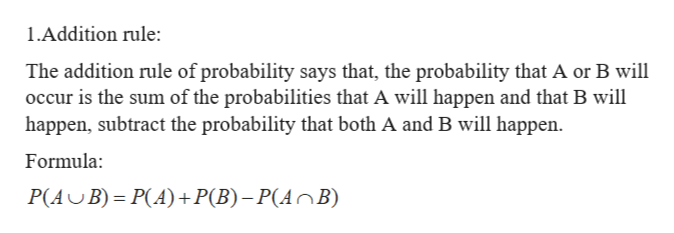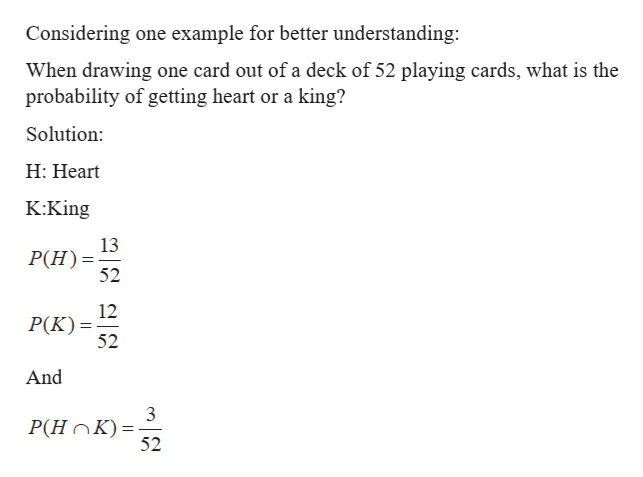# How can I tell whether to use the addition rule or the multiplcation rule when determining probability?

Question

How can I tell whether to use the addition rule or the multiplcation rule when determining probability?

check_circle

Step 1
Step 2help_outlineImage Transcriptionclose1.Addition rule: The addition rule of probability says that, the probability that A or B will occur is the sum of the probabilities that A will happen and that B will happen, subtract the probability that both A and B will happen Formula: P(AB) P(A)+P(B)-P(AnB) fullscreen
Step 3help_outlineImage TranscriptioncloseConsidering one example for better understanding: When drawing one card out of a deck of 52 playing cards, what is the probability of getting heart or a king? Solution: H: Heart K:King 13 P(H) 52 12 P(K) 52 And P(HOK) 52 fullscreen

### Want to see the full answer?

See Solution

#### Want to see this answer and more?

Solutions are written by subject experts who are available 24/7. Questions are typically answered within 1 hour.*

See Solution
*Response times may vary by subject and question.
Tagged in

### Statistics